# Google Summer of Code 2021

Blog## Overview

### Introduction

Welcome!

My name is Lenka, I am currently working on my PhD in Advanced Quantitative Geography at Bristol University , and over the summer 2021 I worked alongside Dr Levi Wolf and Dr Taylor Oshan on implementation of Competing Destination models for SpInt python package, as part of Google Summer of Code 2021.

• If you are future GSoC participant and you are interested in seeing my proposal, it is still living on my google drive.

• To see all the work and commits, see this Github pull request. Or find my working repository here.

• If you want to know how the work progressed step by step, keep reading this blog. Each entry has title, so you can jump to the most recent entry in the table of contents.

• If you are interested what other projects look like, have a look at blogs of Noah and Germano who also completed their projects for Pysal.

### State of the project

I am happy to say that everything stated in proposal has been completed. We have managed to create accessibility function, working test and notebook with an example. We also managed to optimize the function for big datasets and significantly speed up the calculation. Link for the pull request including the whole code is above. The blog documenting tho whole progress is just below, so keep reading if you are interested in that.

### Summary & Final thoughts

We had a lot of discussion about the form of the accessibility. This is a duifficult one as there is many forms and ways it can be calculated, and incorporating them all into this the module would be close to impossible. By defining the function as generally as we did in this project allows the users to interchange wight variables, use different masses and even calculate accessibility for bipartite or tripirtite interaction systems. We hope this will be usefull to many researchers and python users who are looking for Competiong Destination replication.

I loved to be part of GSoC project, as well as the Pysal community. This experience gave me a lot of insight into python developement and especially into the community proccess of developement. This is something that I would not be able to experience otherwise.

## Blog

### 1. Community Bonding, week 24th May till 1th June

#### Meeting the team

On the first meeting, I have been able to connect with my mentors Levi, who is also my PhD supervisor, and Taylor, who is an author of the SpInt package. It has been such a pleasure to connect with Taylor who lives roughly 8.5 thousand kilometres (yes I work in km's and I'm not aiming to change that, so deal with it) away from Bristol where me and Levi are based. We chat about their own experiences with GSoC, as they both have been a participants and now mentors, I was interested in their perspective.
We have also briefly chat about how the SpInt package is structured, so I get better idea of what will the task entail, and what the first steps are.

#### Meeting the Pysal community and other students with their project.

The pysal community has a meeting once a month, where they discuss current problems, updates and future improvements. One of the main things discussed on the meeting was the problem of fragmented graph packages and their inability to cater for translation of graphs from other packages such as street network graphs from osmnx. This is very relevant to my work as I have bumped into this issue in March this year. I was trying to speed up the computation of distances along the road for bunch of points and I found that osmnx has the best function for loading street network based on bounding boxes, but pandana has the fastest computation of shortest paths along the graph (the package is build on C++). To combine these two methods, we just need to translate the graph from osmnx graph to pandana graph. Check out this post to see how this is done and how you can calculate shortest paths using pandana.

It's been good to see how the community takes care of the open-source software, as this part is well hidden from an average user.

### 2. First Coding period. WEEK 1

#### Project introduction

For the general introduction into the project please read the proposal, which provides an overview for the problem.

There is one main deliverable for this project and that is a function that calculate Accessibility term for competing destination models. This can be broken down into X steps.

1. Create initial accessibility function
2. Create functional test to validate the function and its future versions
3. Research how the function could be speeded up

#### What is the accessibility function

According the initial paper from Fotheringham (1983) the accessibility $A_{ij}$ represents the accessibility of destination $j$ to all other destinations available to origin $i$ as perceived by the residents of origin $i$ and is defined as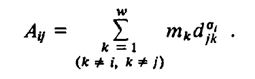This can be little confusing, so let's break it down.

Imagine a migration between 5 following English cities, where people from Liverpool migrate to South Hampton, Bristol and London, and people from London migrate to Brighton. If we want to calculate the accessibility term for the flow Liverpool to Bristol, we consider following; The accessibility of $\text{flow}_{Li,B}$ is the sum of the connections between the destination (Bristol) to all other destinations. This connection or accessibility between Bristol and other destinations can be then calculated as the distance between Bristol and destination times the mass of the destination.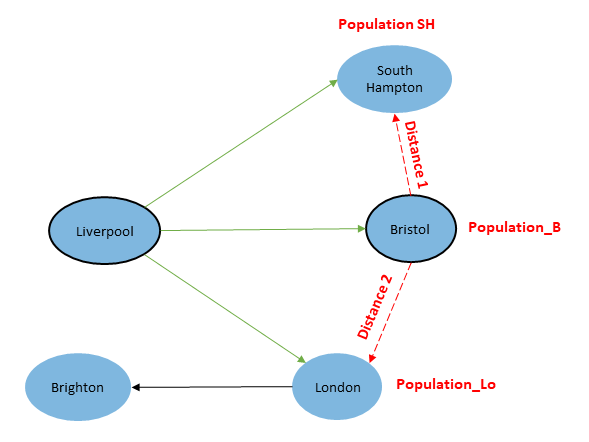In other words;

$$\text{Accessibility for the flow between Liverpool and Bristol} = \sum \text{population SH * distance1, population Lo * distance2}$$

Important: We don't necessarily know if this is meant as an accessibility of all possible destinations in a system, or only those that actually exist for given origin.

I could design 2 functions

1. Accessibility of flow taking all potential destinations in the system
2. Accessibility of flow taking only existing destinations


In those two functions, we would assume that the input is just one Data frame: Data Frame of all possible flows in system with distances between them, masses on destinations and the flow volumes between existing flows (0 where no flow exists). First I draw the second function which accounts only for those connections that exists in flow network.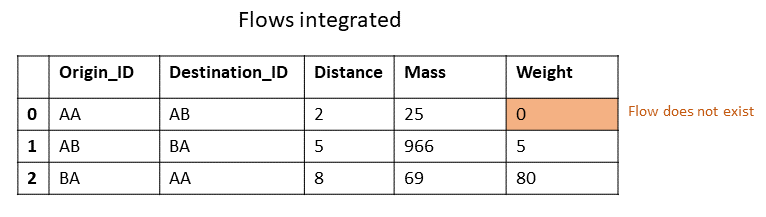#### Initial accessibility function

# coding=utf-8
# 3. AFED = Accessibility of flow taking existing destinations

def AFED(flow_df, row_index): # AFAPF

# rename teh columns so we can call them
flow_df = flow_df.rename(columns = {flow_df.columns:'origin_ID',
flow_df.columns:'dest_ID',
flow_df.columns:'dist',
flow_df.columns:'weight',
flow_df.columns:'dest_mass'})
# define O and D for each row the variables
D = flow_df['dest_ID'][row_index]
O = flow_df['origin_ID'][row_index]

# get the list of possible destinations
all_dest = (flow_df.query('origin_ID == @O')
.query('weight > 0')
['dest_ID']
.unique()
)

# Create all destination flows
x1 = pd.DataFrame({'D': np.array([D]*len(all_dest), dtype=object),
'dests':all_dest}).merge(flow_df, how='left', left_on=['D','dests'], right_on=['origin_ID','dest_ID'])

# merge with the distances and masses

# Delete the flow to origin
x1 = x1[~x1.dests.isin(list(O))]

# calculate the accessibility
A = (x1['dist']*x1['dest_mass']).sum()

return A


#### Further notes on accessibility function

I have described accessibility function defined by Fotheringham (1983) as very generic function of distances and masses, however, more specific definition with different weighting could be considered for specific situations. For example, some could opt to substitute distance for some more specific measure of separation, such as travel time or journey cost. Similarly, there is many things that could be considered as a mass on the destination.

Note: it would be good to discuss the potential of the function to cater for alternative definitions of accessibility

### 3. First Coding period. WEEK 2 and 3

#### Extending the accessibility calculation, opt for all possible connections or just existing connections

As I said last week (few lines above), you can consider either all potential destinations or the existing ones.

Let me clarify this a bit. Estimating accessibility in a very generic way that we do here, we do in 3 steps.

For each unique combination of origin A and destination B;

1. find all the potential or all existing destinations to origin A
2. find the distances between all those destinations and destination B
3. compute (distance*destination mass) for all those connections from B to other destinations
4. deduct the (distance*destination mass) which belongs to destination B and origin A

In point 1, all potential destinations are those that exist in a Spatial Interaction system. Even though there is no flow between our origin A and destination X, it is available for people from Origin A.

However, if there is no flow between origin A and destination X in a system, then we could assume that people from that origin just do not consider that destination desirable. Thus, as competing destinations we could consider these, that receive at least some flow from origin A (flow volume > 0).

Have a look at this example:In the updated function, I include an if statement which either constructs the competing destinations based on all potential flows or just the existing ones. This is controllable by the argument all_destination = False, where False (include only the existing flows) is the default option, while True will include all the potential destinations regardless of the flow volume.

# coding=utf-8
# 3. FlowAccessibility = Accessibility of flow taking existing destinations

def AFED(flow_df, row_index, all_destinations=False):

# rename teh columns so we can call them
flow_df = flow_df.rename(columns = {flow_df.columns:'origin_ID',
flow_df.columns:'dest_ID',
flow_df.columns:'dist',
flow_df.columns:'weight',
flow_df.columns:'dest_mass'})
# define O and D for each row the variables
D = flow_df['dest_ID'][row_index]
O = flow_df['origin_ID'][row_index]

# get the list of possible destinations
if all_destinations:
all_dest = (flow_df.query('origin_ID == @O')
['dest_ID']
.unique()
)
else:
all_dest = (flow_df.query('origin_ID == @O')
.query('weight > 0')
['dest_ID']
.unique()
)

# Create all destination flows
x1 = pd.DataFrame({'D': np.array([D]*len(all_dest), dtype=object),
'dests':all_dest}).merge(flow_df, how='left', left_on=['D','dests'], right_on=['origin_ID','dest_ID'])

# merge with the distances and masses

# Delete the flow to origin
x1 = x1[~x1.dests.isin(list(O))]

# calculate the accessibility
A = (x1['dist']*x1['dest_mass']).sum()

return A


#### Application of Accessibility function for whole dataset

The function defined above calculates accessibility for a one row of data, for one connection in the interaction system. In next step, I need to do this for all rows in the dataset. This is probably not the most efficient way, but we need to start somewhere.

Below, I define another function that takes the accessibility computation, loops it over the full dataset supplied, and tells you how long it took.

def Accessibility(flow_df, function, all_destinations=False):
start = timer()

A_ij = []

for idx in flow_df.index:

if all_destinations:
A = function(flow_df=flow_df, row_index=idx, all_destinations=True)
else:
A = function(flow_df=flow_df, row_index=idx, all_destinations=False)
A_ij.append(A)

A_ij = pd.Series(A_ij)
end = timer()

print('time elapsed: ' + str(end - start))
return A_ij


#### Contributing to GitHub

So, now is the time to get these online into a repo!

If you are not familiar with a GitHub yet, here are some useful links

1. Here is a video with the most effective explanation of what is GitHub.
2. This video explains and demonstrates forking, branching merging, pull requests and even more. I like this video and the presenter because he is very humanly and slightly quirky.
3. On the same channel, you can also find branching video and [Intro to GitHub video](](https://www.youtube.com/watch?v=_NrSWLQsDL4&t=491s).
4. This video provides a tutorial for the command line fundamentals. You can find more specific step-by-step guide here.

Lastly, here is a cheat-sheet that I created for myself. I am one of these people who do not hold in memory things that I can easily find online, however, that can be sometimes a bit lengthy. So, cheat-sheets are the best way I can quickly access things that I already did, but don't hold them in memory.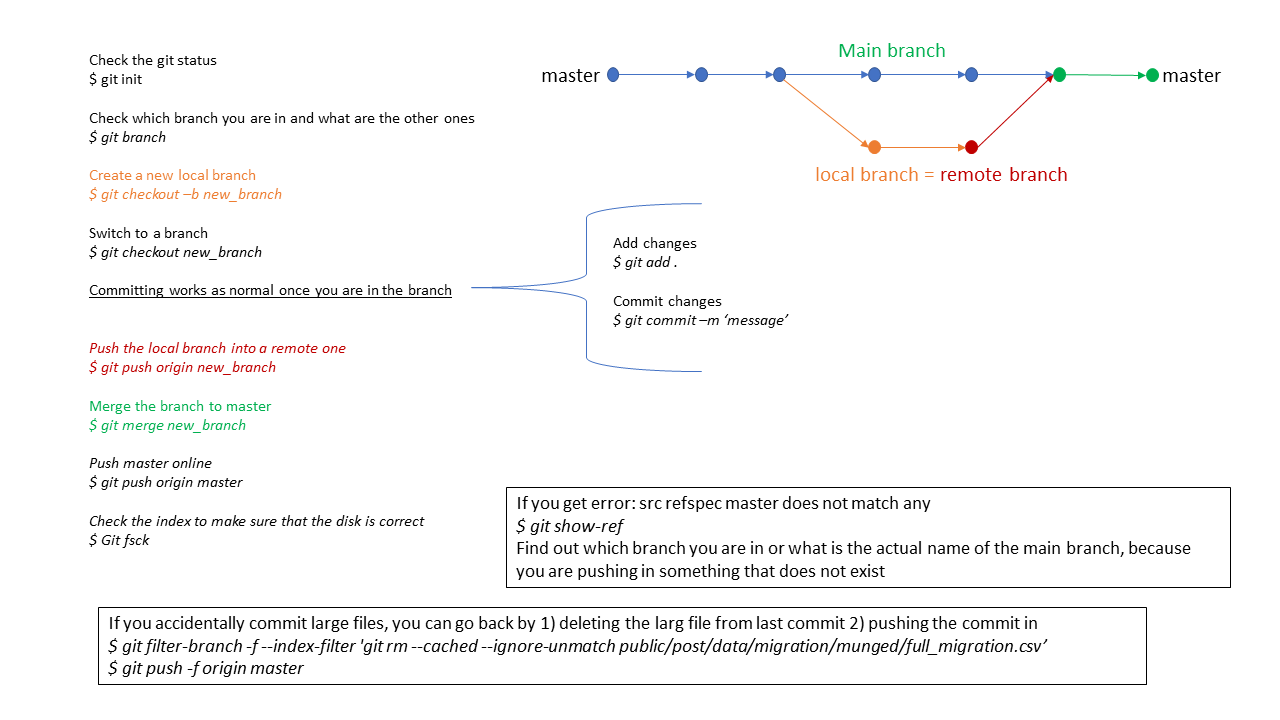To contribute to a package, I did this;

1. fork the pysal/spint package = create copied-repository on my own GitHub
2. commit changes, new codes and fixes into this repo over the period of this project
3. once this project is getting to the end, and everybody is happy with the contribution, I will create pull request to send the code from my repo to the official pysal repo.

### 4. First Coding period. WEEK 4 and 5

#### Testing and validation

the next step is to create an efficient test, which will be automatically checking if the version of the function in the package is correct. To do this, I need to;

1. create a simple dummy dataset for which I manually calculate the desired accessibility values
2. create test_accessibility.py script that will use the function from the package to calculate accessibility for dummy data and check if it equals to manually calculated values.
3. make sure this test runs correctly within the framework

#### Create dummy dataset

The simplest way to test if function works is to see if its output matches the expected output. Nothing scary, nothing revolutionary. Simple yet effective.

Below you can see simple data set that I use for testing. It only has 5 points in space, but it has all possible types of connections and has realistic masses and distances.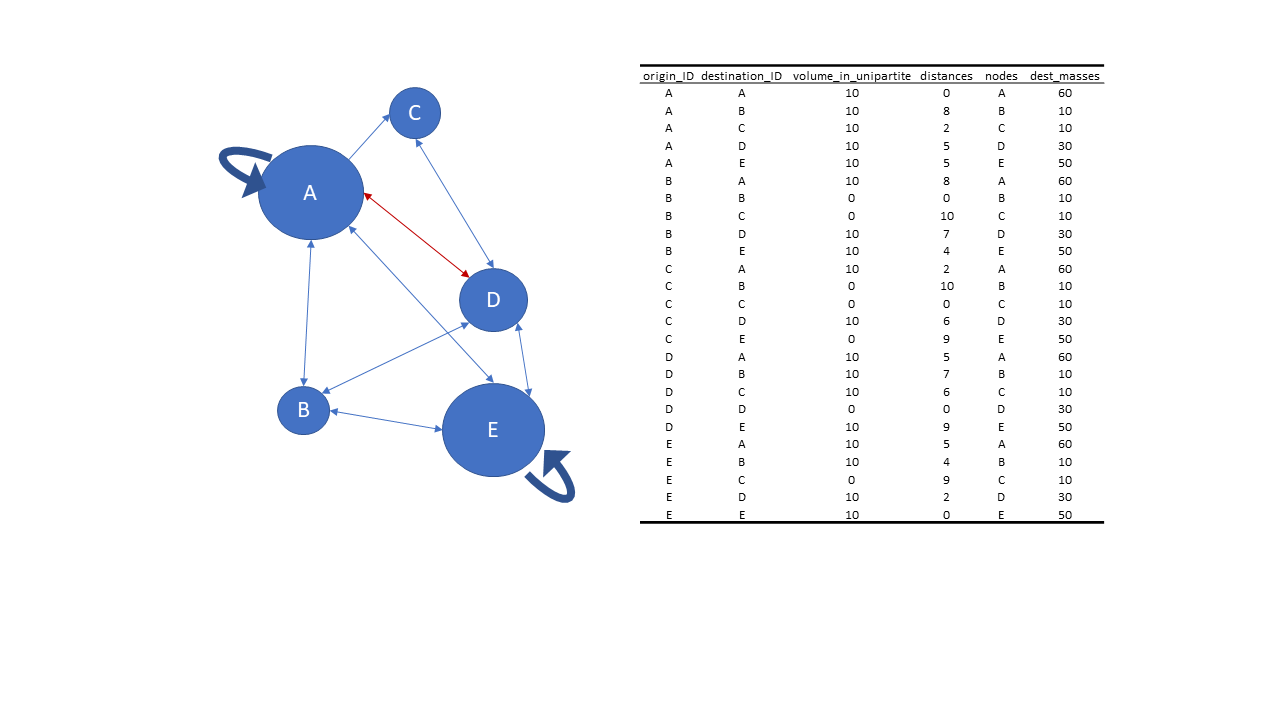#### Create test

I was not familiar with testing in python until now, so I needed to do some studying for this. I found these two links very useful;

There are two modules used for testing in python; pytest and unittest. Pytest is the newer one and is super easy to use. Check out this quick tutorial. While unittest is the more established module, that is already used within the pysal/spint.

"""
Tests for Accessibility function.

The correctness of the function is veryfied by matching the output to manually calculated values, using very simple dummy dataset.

"""

import unittest
import numpy as np
from generate_dummy import generate_dummy_flows # this is a function that generates dummy flow
from ..flow_accessibility import Accessibility # This is the function that applies accessibility to whole dataset
from ..flow_accessibility import AFED # Accessibility function

class AccessibilityTest(unittest.TestCase):

def test_accessibility(self):
flow = generate_dummy_flows()
flow = flow.loc[:,['origin_ID', 'destination_ID','distances', 'volume_in_unipartite','dest_masses','results_all=False']]
flow['acc_uni'] = Accessibility(flow_df = flow, all_destinations=False, function = AFED)

self.assertEqual(flow['results_all=False'].all(), flow['acc_uni'].all())

if __name__ == '__main__':
unittest.main()



To run the test on your local machine, just open your anaconda prompt, navigate to directory where all the tests are and run following

$python -m unittest test_accessibility.py Or simply just push the code online and login to Travis.Cl. Travis runs all the test every time changes are made in your repo. Don't forget to signup to Travis and connect it to your GitHub. So cool! ### 5. What's next? With the test that works, most of the important work on this project is basically ready to be submitted. However, because the function compromises 2 for loops, it is more likely to be very slow when you apply it on the big data. In real word research, you don't just have 5 points in space that creates$5*5=25\$ flows. Often you have tens, hundreds and even thousands of points. For example, if you would have to compute accessibility for all the flows between all UK train stations (there is 2,563 of them) you would have 6,568,969 flows.

The rest of the GSoC project I will dedicate to trying to find out how fast is the current function, what is the best way to speed it up and how that can be implemented in practice.

### 6. Second coding period, making the code faster

The function I wrote initially can be quite slow. We can already tell that from the code, where we use for loop to calculate accessibility for each row of your dataset. for loops are not very efficient way to compute things, unless you have very few jobs.

There are few ways the speed up your code. Here are the two most appropriate options:

If I would really need the loops, Numba is a really good way to speed it up by allowing for parralel processing. But sometimes the loops are not that necessary and can be rewriten more efficiently using arrays and matrices. So lets try to do that!

The code bellows shows you 3 functions and compares their speed on real data (set of points located within Bristol).

1. orginal function from above (AFED)
2. function that loops over set of arrays (AFED_MAT)
3. function that multiplies 3-d arrays (no loops) (AFED_MAT_2)
import numpy as np
import pandas as pd
import geopandas as gpd
import itertools
import libpysal
from timeit import default_timer as timer
import matplotlib.pyplot as plt

from geopy.distance import geodesic, great_circle
from pyproj import CRS


#### Define the functions

def AFED(flow_df, row_index, all_destinations=False):

# rename teh columns so we can call them
flow_df = flow_df.rename(columns = {flow_df.columns:'origin_ID',
flow_df.columns:'dest_ID',
flow_df.columns:'dist',
flow_df.columns:'weight',
flow_df.columns:'dest_mass'})

flow_df['dist'] = flow_df['dist'].astype(int)
flow_df['weight'] = flow_df['weight'].astype(int)
flow_df['dest_mass'] = flow_df['dest_mass'].astype(int)

# define O and D for each row the variables
D = flow_df['dest_ID'][row_index]
O = flow_df['origin_ID'][row_index]

# get the list of possible destinations
if all_destinations:
all_dest = (flow_df.query('origin_ID == @O')
['dest_ID']
.unique()
)
else:
all_dest = (flow_df.query('origin_ID == @O')
.query('weight > 0')
['dest_ID']
.unique()
)

# Create all destination flows
x1 = pd.DataFrame({'D': np.array([D]*len(all_dest), dtype=object
), 'dests':all_dest}
).merge(flow_df, how='left', left_on=['D','dests'], right_on=['origin_ID','dest_ID'])

# merge with the distances and masses

# Delete the flow to origin
x1 = x1[~x1.dests.isin([O])]

# calculate the accessibility
A = (x1['dist']*x1['dest_mass']).sum()

return A


import numpy as np
import pandas as pd
from timeit import default_timer as timer
import itertools

def AFED_MAT(flow_df, all_destinations=False):

# rename teh columns so we can call them
flow_df = flow_df.rename(columns = {flow_df.columns:'origin_ID',
flow_df.columns:'dest_ID',
flow_df.columns:'dist',
flow_df.columns:'weight',
flow_df.columns:'dest_mass'})

flow_df['dist'] = flow_df['dist'].astype(int)
flow_df['weight'] = flow_df['weight'].astype(int)
flow_df['dest_mass'] = flow_df['dest_mass'].astype(int)

# create binary for weight
flow_df['v_bin'] = 1
flow_df['v_bin'][(flow_df['weight'].isna() | flow_df['weight'] == 0 ) ] = 0

# define base matrices
distance = flow_df.pivot_table(values='dist', index='dest_ID', columns="origin_ID")
mass = flow_df.pivot_table(values='dest_mass', columns='dest_ID', index="origin_ID")
exists = flow_df.pivot_table(values='v_bin', index='dest_ID', columns="origin_ID")

# lists of O-D
list_c = list(itertools.product(exists.columns,exists.index))
df = pd.DataFrame(list_c).rename(columns = {0:'origin_ID', 1:'dest_ID'})

# Start function
array = []
start = timer()
for i,j in list_c:

if all_destinations:
f = exists.loc[i,:]
f =  1
f[f.index.isin([i])] = 0
else:
f = exists.loc[i,:]
f[f.index.isin([i])] = 0

empty = pd.DataFrame(np.zeros((len(exists.index),len(exists.columns))), index = exists.index, columns = exists.columns)
empty.loc[j,:] = f
A_ij = empty *  (distance * mass)
A_ij = A_ij.sum().sum()
array.append(A_ij)

# Get the result back to original dataframe
df['A_ij'] = array
flow_df = flow_df.merge(df, how = 'left', on = ['origin_ID','dest_ID'])

#how long did this took?
end = timer()
print('time elapsed: ' + str(end - start))
return(flow_df)


def AFED_VECTOR(flow_df, all_destinations=False):
start = timer()
# rename teh columns so we can call them
flow_df = flow_df.rename(columns = {flow_df.columns:'origin_ID',
flow_df.columns:'dest_ID',
flow_df.columns:'dist',
flow_df.columns:'weight',
flow_df.columns:'dest_mass'})

flow_df['dist'] = flow_df['dist'].astype(int)
flow_df['weight'] = flow_df['weight'].astype(int)
flow_df['dest_mass'] = flow_df['dest_mass'].astype(int)
# create binary for weight
flow_df['v_bin'] = 1
flow_df.loc[flow_df['weight'].isna(),'v_bin'] = 0
flow_df.loc[flow_df['weight'] <= 0,'v_bin'] = 0

# define the base matrices
distance = np.array(flow_df.pivot_table(values='dist', index='origin_ID', columns="dest_ID"))
mass = np.array(flow_df.pivot_table(values='dest_mass', columns="origin_ID", index='dest_ID'))
exists = np.array(flow_df.pivot_table(values='v_bin', index='dest_ID', columns="origin_ID"))

# define the base 3d array
nrows= len(exists)
ones = np.ones((nrows,len(flow_df.origin_ID.unique()),len(flow_df.dest_ID.unique())))

# define the identity array
idn = np.identity(nrows)
idn = np.where((idn==1), 0, 1)
idn = np.concatenate(nrows * [idn.ravel()], axis = 0).reshape(nrows,nrows,nrows).T

# multiply the distance by mass
ard = np.array(distance)*np.array(mass)

# combine all into and calculate the output

if all_destinations:
output = np.array(idn) * (np.array(nrows * [ard]
)
)

else:
output = (np.concatenate(nrows * [exists], axis = 0
).reshape(nrows,nrows,nrows
).T
) * np.array(idn) * (np.array(nrows * [ard]
)
)

# get the sum and covert to series
g = pd.DataFrame((
np.sum(output,axis = 1
)  ).reshape(1,len(flow_df)
).T
)

end = timer()
print('time elapsed: ' + str(end - start))
return g


#### Define the data sets for testing

pois = gpd.read_file("./../points.geojson")
pois.info()

<class 'geopandas.geodataframe.GeoDataFrame'>
RangeIndex: 855 entries, 0 to 854
Data columns (total 4 columns):
#   Column    Non-Null Count  Dtype
---  ------    --------------  -----
0   ID_code   855 non-null    object
1   X         855 non-null    float64
2   Y         855 non-null    float64
3   geometry  855 non-null    geometry
dtypes: float64(2), geometry(1), object(1)
memory usage: 26.8+ KB

# strip
pois_all = pois.iloc[:,[0,3]]

pois_all['mass'] = pd.Series( np.random.randint(0,1500, size=len(pois_all))
)
# create stripped column of coordinates
pois_all['xy'] = pois_all.geometry.apply(lambda x: [x.y, x.x])

# get all unique combinations of all the origins and destinations
flow_all = pd.DataFrame( list( itertools.product( pois_all['ID_code'].unique(),pois_all['ID_code'].unique())
)
).rename(columns = {0:'origin',
1:'destination'})

# joining the xy to flows and create distances
distances_all = flow_all.merge(pois_all.loc[:,['ID_code','xy']], how = 'left', left_on = 'origin', right_on = 'ID_code'
).merge(pois_all.loc[:,['ID_code','xy']], how = 'left', left_on = 'destination', right_on = 'ID_code'
)

# calculate distances
distances_all['great_circle_dist'] = distances_all.apply(lambda x: great_circle(x.xy_x, x.xy_y).km, axis=1)
distances_all = distances_all.loc[:,['origin','destination','great_circle_dist']]

# list points
list5 = list(pois_all['ID_code'][0:6])
list10 = list(pois_all['ID_code'][0:11])
list20 = list(pois_all['ID_code'][0:21])
list40 = list(pois_all['ID_code'][0:41])
list80 = list(pois_all['ID_code'][0:81])
list160 = list(pois_all['ID_code'][0:161])

# Define different number of points
pois_all_s = pd.DataFrame(pois_all.loc[:,['ID_code','mass']])
pois5 = pois_all_s[pois_all_s['ID_code'].isin(list5)]
pois10 = pois_all_s[pois_all_s['ID_code'].isin(list10)]
pois20 = pois_all_s[pois_all_s['ID_code'].isin(list20)]
pois40 = pois_all_s[pois_all_s['ID_code'].isin(list40)]
pois80 = pois_all_s[pois_all_s['ID_code'].isin(list80)]
pois160 = pois_all_s[pois_all_s['ID_code'].isin(list160)]

flow5 = flow_all[flow_all['origin'].isin(list5) & flow_all['destination'].isin(list5)].reset_index(drop=True)
flow10 = flow_all[flow_all['origin'].isin(list10) & flow_all['destination'].isin(list10)].reset_index(drop=True)
flow20 = flow_all[flow_all['origin'].isin(list20) & flow_all['destination'].isin(list20)].reset_index(drop=True)
flow40 = flow_all[flow_all['origin'].isin(list40) & flow_all['destination'].isin(list40)].reset_index(drop=True)
flow80 = flow_all[flow_all['origin'].isin(list80) & flow_all['destination'].isin(list80)].reset_index(drop=True)
flow160 = flow_all[flow_all['origin'].isin(list160) & flow_all['destination'].isin(list160)].reset_index(drop=True)

distances5 = distances_all[distances_all['origin'].isin(list5) & distances_all['destination'].isin(list5)].reset_index(drop=True)
distances10 = distances_all[distances_all['origin'].isin(list10) & distances_all['destination'].isin(list10)].reset_index(drop=True)
distances20 = distances_all[distances_all['origin'].isin(list20) & distances_all['destination'].isin(list20)].reset_index(drop=True)
distances40 = distances_all[distances_all['origin'].isin(list40) & distances_all['destination'].isin(list40)].reset_index(drop=True)
distances80 = distances_all[distances_all['origin'].isin(list80) & distances_all['destination'].isin(list80)].reset_index(drop=True)
distances160 = distances_all[distances_all['origin'].isin(list160) & distances_all['destination'].isin(list160)].reset_index(drop=True)

li_ommited = [1,4,5,9,13,15,17,26,27,28,34]

flow5_ommited = flow5.drop(flow5.index[li_ommited]).reset_index(drop=True)
flow10_ommited = flow10.drop(flow10.index[li_ommited]).reset_index(drop=True)
flow20_ommited = flow20.drop(flow20.index[li_ommited]).reset_index(drop=True)
flow40_ommited = flow40.drop(flow40.index[li_ommited]).reset_index(drop=True)
flow80_ommited = flow80.drop(flow80.index[li_ommited]).reset_index(drop=True)
flow160_ommited = flow160.drop(flow160.index[li_ommited]).reset_index(drop=True)

# combine the points and distances
flow_integrated = distances_all.merge(pois_all_s, how = 'left', left_on=['destination'], right_on=['ID_code'])

#generate some weights
flow_integrated['weight'] = pd.Series( np.random.randint(10,1500, size=len(flow_integrated)) # here - and 0 represent flows that does not exist
)
# reorder
flow_integrated = flow_integrated.loc[:,['origin','destination','great_circle_dist','weight','mass']]

# build short datasets
flow5_integrated = flow_integrated[flow_integrated['origin'].isin(list5) & flow_integrated['destination'].isin(list5)].reset_index(drop=True)
flow10_integrated = flow_integrated[flow_integrated['origin'].isin(list10) & flow_integrated['destination'].isin(list10)].reset_index(drop=True)
flow20_integrated = flow_integrated[flow_integrated['origin'].isin(list20) & flow_integrated['destination'].isin(list20)].reset_index(drop=True)
flow40_integrated = flow_integrated[flow_integrated['origin'].isin(list40) & flow_integrated['destination'].isin(list40)].reset_index(drop=True)
flow80_integrated = flow_integrated[flow_integrated['origin'].isin(list80) & flow_integrated['destination'].isin(list80)].reset_index(drop=True)
flow160_integrated = flow_integrated[flow_integrated['origin'].isin(list160) & flow_integrated['destination'].isin(list160)].reset_index(drop=True)

flow5_integrated['great_circle_dist'] = round(flow5_integrated['great_circle_dist'],0)
flow10_integrated['great_circle_dist'] = round(flow10_integrated['great_circle_dist'],0)
flow20_integrated['great_circle_dist'] = round(flow20_integrated['great_circle_dist'],0)
flow40_integrated['great_circle_dist'] = round(flow40_integrated['great_circle_dist'],0)
flow80_integrated['great_circle_dist'] = round(flow80_integrated['great_circle_dist'],0)
flow160_integrated['great_circle_dist'] = round(flow160_integrated['great_circle_dist'],0)



origin destination great_circle_dist weight mass
0 A91120 A91120 0.0 906 1039
1 A91120 A99931 5.0 585 993
2 A91120 A99986 3.0 264 600
3 A91120 L81002 30.0 1495 1408
4 A91120 L81004 15.0 1135 762

#### Apply the functions


# for 05 dataset function 3
list_AFED = []

# 5
start = timer()
A_ij = []
for idx in flow5_integrated.index:
A = AFED(flow_df=flow5_integrated, row_index=idx)
A_ij.append(A)
end = timer()
t = (end - start)
list_AFED.append(t)

# 10
start = timer()
A_ij = []
for idx in flow10_integrated.index:
A = AFED(flow_df=flow10_integrated, row_index=idx)
A_ij.append(A)
end = timer()
t = (end - start)
list_AFED.append(t)

# 20
start = timer()
A_ij = []
for idx in flow20_integrated.index:
A = AFED(flow_df=flow20_integrated, row_index=idx)
A_ij.append(A)
end = timer()
t = (end - start)
list_AFED.append(t)

# 40
start = timer()
A_ij = []
for idx in flow40_integrated.index:
A = AFED(flow_df=flow40_integrated, row_index=idx)
A_ij.append(A)
end = timer()
t = (end - start)
list_AFED.append(t)

# 80
start = timer()
A_ij = []
for idx in flow80_integrated.index:
A = AFED(flow_df=flow80_integrated, row_index=idx)
A_ij.append(A)
end = timer()
t = (end - start)
list_AFED.append(t)

# 160
start = timer()
A_ij = []
for idx in flow160_integrated.index:
A = AFED(flow_df=flow160_integrated, row_index=idx)
A_ij.append(A)
end = timer()
t = (end - start)
list_AFED.append(t)

list_AFED

[0.26563450000000444,
0.8232417000000112,
3.175610599999999,
12.360262700000007,
54.0198017,
303.65919140000005]


# for 05 dataset function 3
list_AFED_MAT = []

# 5
start = timer()
flow5_mat = AFED_MAT(flow5_integrated)
end = timer()
t = (end - start)
list_AFED_MAT.append(t)

# 10
start = timer()
A_ij = []
flow10_mat = AFED_MAT(flow10_integrated)
end = timer()
t = (end - start)
list_AFED_MAT.append(t)

# 20
start = timer()
A_ij = []
flow20_mat = AFED_MAT(flow20_integrated)
end = timer()
t = (end - start)
list_AFED_MAT.append(t)

# 40
start = timer()
A_ij = []
flow40_mat = AFED_MAT(flow40_integrated)
end = timer()
t = (end - start)
list_AFED_MAT.append(t)

# 80
start = timer()
A_ij = []
flow80_mat = AFED_MAT(flow80_integrated)
end = timer()
t = (end - start)
list_AFED_MAT.append(t)

# 160
start = timer()
A_ij = []
flow160_mat = AFED_MAT(flow160_integrated)
end = timer()
t = (end - start)
list_AFED_MAT.append(t)

time elapsed: 0.06575129999998808
time elapsed: 0.2165177999999628
time elapsed: 0.8499411000000237
time elapsed: 3.23107200000004
time elapsed: 14.276894200000015
time elapsed: 93.39352159999999


# for 05 dataset function 3
list_AFED_VECTOR = []

# 5
start = timer()
flow5_mat = AFED_VECTOR(flow5_integrated)
end = timer()
t = (end - start)
list_AFED_VECTOR.append(t)

# 10
start = timer()
A_ij = []
flow10_mat = AFED_VECTOR(flow10_integrated)
end = timer()
t = (end - start)
list_AFED_VECTOR.append(t)

# 20
start = timer()
A_ij = []
flow20_mat = AFED_VECTOR(flow20_integrated)
end = timer()
t = (end - start)
list_AFED_VECTOR.append(t)

# 40
start = timer()
A_ij = []
flow40_mat = AFED_VECTOR(flow40_integrated)
end = timer()
t = (end - start)
list_AFED_VECTOR.append(t)

# 80
start = timer()
A_ij = []
flow80_mat = AFED_VECTOR(flow80_integrated)
end = timer()
t = (end - start)
list_AFED_VECTOR.append(t)

# 160
start = timer()
A_ij = []
flow160_mat = AFED_VECTOR(flow160_integrated)
end = timer()
t = (end - start)
list_AFED_VECTOR.append(t)

time elapsed: 0.023291800000038165
time elapsed: 0.023622900000077607
time elapsed: 0.022046499999987645
time elapsed: 0.026168299999994815
time elapsed: 0.04007190000004357
time elapsed: 0.15891480000004776


#### Evaluate

times = pd.DataFrame({'original': pd.Series(list_AFED),
'matrices': pd.Series(list_AFED_MAT),
'vectorized': pd.Series(list_AFED_VECTOR)})
times


original matrices vectorized
0 0.265635 0.093522 0.023567
1 0.823242 0.240578 0.024067
2 3.175611 0.879755 0.022347
3 12.360263 3.258259 0.026568
4 54.019802 14.310321 0.041264
5 303.659191 93.476836 0.166047
fig, ax = plt.subplots(figsize=(15, 10))
(times.iloc[:,0:3]/60).plot(ax = ax,  linewidth=5)
plt.xlabel("Number of observations/flows",size=15)
plt.ylabel("Minutes",size=15)
plt.xticks(times.index, (str(len(flow5)), str(len(flow10)), str(len(flow20)), str(len(flow40)),str(len(flow80)), str(len(flow160))),size=15)
plt.yticks(size=15)
plt.legend( prop={'size': 15})
plt.show();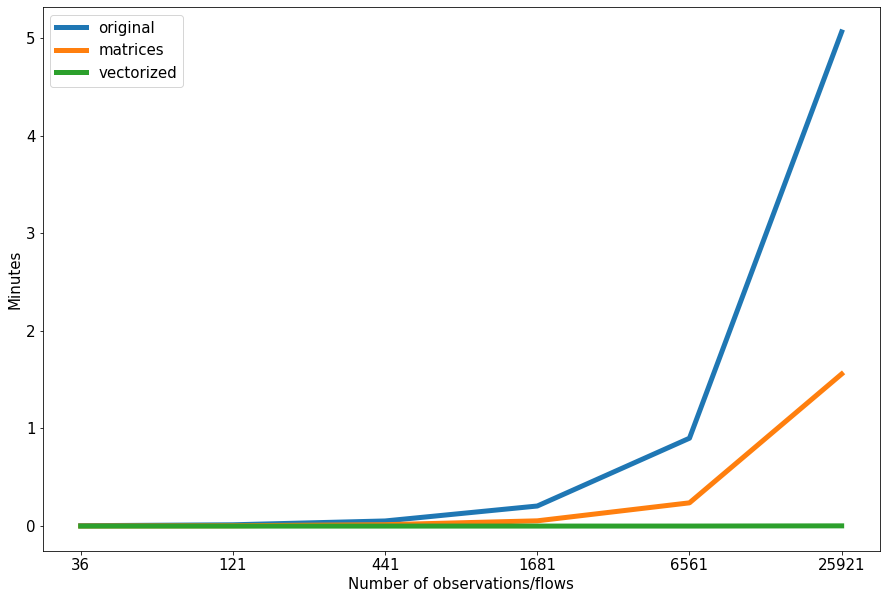From the graph above you can see that with each new finction the speed improved. There is very little difference in the speed if your data has less then 200 observations/flows (or 14 origins and destinations). However, when your data sets are bigger, the speed becomes significantly better with the vectorized function, then the original one. For example, 25,921 observations/flows (161 origins and destinations will take only 17 seconds to compute by vectorized function, in compare with the original one that takes close to 6 minutes to compute.

### 7. Changes after review

After the mentors reviewed my code, there was few places for improvements.

• Instead of feeding dataframe in, we can just feed numpy arrays. This simply makes things easier for the user as less thing can go wrong with the input.
• We can create an error message which checks that all the inputs are the same length. This will validate that the input is correct.
• There is also some unnecessary stuff to delete.
• The test could use more appropriate function.

#### Final version of function

def Accessibility(nodes, distances, weights, masses, all_destinations=False):

# convert numbers to integers
distances = np.array(distances.astype(int))
weights = np.array(weights.astype(int))
masses = np.array(masses.astype(int))
nodes = np.array(nodes)

# define error
if len(distances) != len(weights) != len(masses) != len(nodes):
raise ValueError("One of the input array is different length then the others, but they should all be the same length. See notebook example if you are unsure what the input should look like ")

# define number of rows
nrows= len(nodes)
uniques = len(np.unique(np.array(nodes)))

# create binary for weight
v_bin =  np.ones(nrows)
weights[np.isnan(weights)] = 0
v_bin[weights <= 0] = 0

# define the base matrices
distance = distances.reshape(uniques,uniques)
mass =masses.reshape(uniques,uniques).T
exists = v_bin.reshape(uniques,uniques)

# define the identity array
idn = np.identity(uniques)
idn = np.where((idn==1), 0, 1)
idn = np.concatenate(uniques * [idn.ravel()], axis = 0
).reshape(uniques,uniques,uniques
).T

# multiply the distance by mass
dm = distance * mass

# combine all matrices for either all or existing destinations
if all_destinations:
output = idn * (nrows * [dm])

else:
output = (np.concatenate(uniques * [exists], axis = 0
).reshape(uniques,uniques,uniques
).T
) * idn * (uniques * [dm]
)

# get the sum and covert to series
output = (np.sum(output,axis = 1
)
).reshape(nrows
).T

return output



#### Final version of test

class AccessibilityTest(unittest.TestCase):

def test_accessibility(self):
flow = _generate_dummy_flows()
flow = flow.loc[:,['origin_ID', 'destination_ID','distances', 'volume_in_unipartite','dest_masses','results_all=False']]
flow['acc_uni'] = Accessibility(nodes = flow['origin_ID'],  distances = flow['distances'], weights = flow['volume_in_unipartite'], masses = flow['dest_masses'], all_destinations=False)

np.testing.assert_array_equal(flow['results_all=False'].all(), flow['acc_uni'].all())

if __name__ == '__main__':
unittest.main()